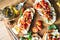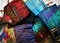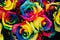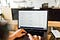# Partial Least Squares

## A deep-dive into Partial Least Squares Regression and Partial Least Squares Discriminant Analysis with full worked-out examples in R and PythonPartial Least Squares — Applied to an Olive Oil and a Meat example — Photo by Calum Lewis on Unsplash

# Multidimensional Scaling

• Metric Multidimensional Scaling, also called Principal Coordinate Analysis, is a subtype of Multidimensional Scaling that deals with numerical distances, in which there is no measurement error (you have exactly one distance measure for each pair of items).
• Nonmetric Multidimensional Scaling is a subcategory of Multidimensional Scaling that deals with non-numerical distances between items, in which there is no measurement error (you have exactly one distance measure for each pair of items).

# Canonical Correlation Analysis

• Focusing on a dependence relationship, and model the two datasets in a regression-like manner: data set y as a function of data set x.
• Focusing on the exploration of the relationships between the two datasets without stating any data set as the dependent or the independent variables. …

# Structural Equation Modeling

## A comprehensive overview of Structural Equation Modeling with a full walkthrough of an example using both R and PythonStructural Equation Modeling — Photo by RhondaK Native Florida Folk Artist on Unsplash

# What is Structural Equation Modeling?

• measuring a score on Multiple Choice questionnaires in multiple domains
• converting those scores into an estimate of Intelligence

# Assumptions of linear regression

## With implementations in R and PythonAssumptions of linear regression — Photo by Denise Chan on Unsplash

# What is the difference between PCA and Factor Analysis?

## Learn the difference between PCA and Factor Analysis — and when to use which — with Python and R example codeWhat is the difference between PCA and Factor Analysis? Photo by Austin Distel on Unsplash.

# Multivariate Statistics — Reducing the number of variables

Multivariate Statistics deals with the treatment of data sets with a large number of dimensions.

# Waiting Line Models

## A Full Guide to Waiting Line Models and Queuing Theory